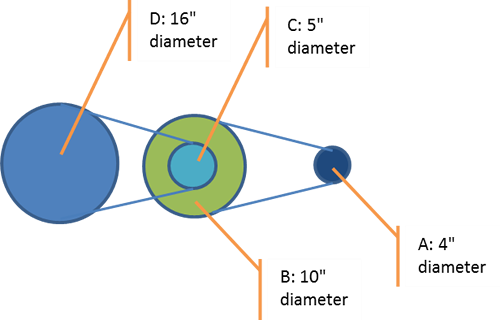A larger pulley causes a smaller pulley to rotate faster by the ratio of their diameters. If pulley A rotates at 100 rpm, then pulley B rotates at $$\frac{\mathrm{100 \times 4} }{\mathrm{10} } = 40$$ rpm. Since C is directly connected to B it rotates at the same rate (40 rpm). Finally, pulley D will rotate at $$40 \times \frac{\mathrm{5} }{\mathrm{16} } = 12.5$$ rpm.